## Stata

SYSTECH is AUTHORISED DISTRIBUTOR for STATA Software Products and solution in INDIA

Read about all the new features in Stata 14 below. And, these are just the new features. You can learn about all of Stata and about all of Stata's features.

### IRT (item response theory)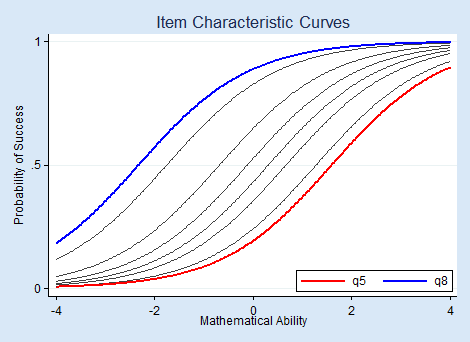• Binary response models&mdash-1PL, 2PL, 3PL
• Ordinal response models&mdash-graded response, partial credit, rating scale
• Nominal response model
• Hybrid models
• Item characteristic curves
• Test characteristic curves
• Item information function
• More

### Unicode• Data
• Variable and value labels
• Variable names!
• More

### Integration with Excel• Cell formatting
• Font formatting
• Insert Stata graphs
• Create cell formulas

### Treatment effects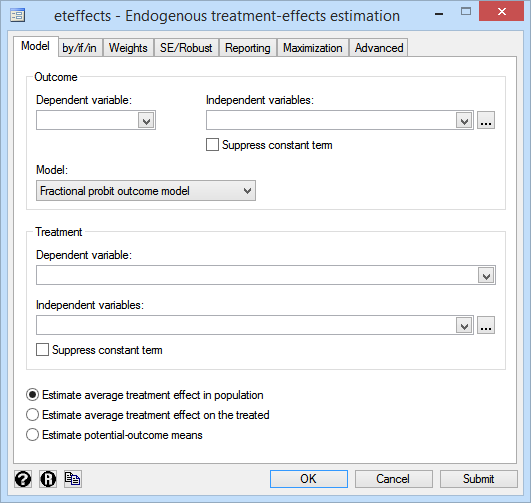### Multilevel survival models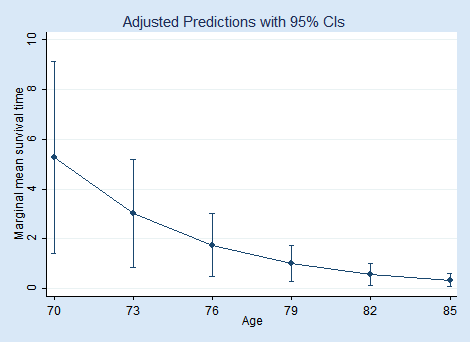• Random effects
• Crossed effects
• Two, three, higher level
• Right censoring
• Exponential, Weibull, ...
• Survey data

### Multilevel models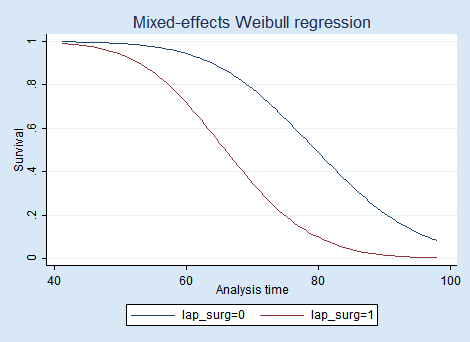### SEM (structural equation modeling)### Power and sample size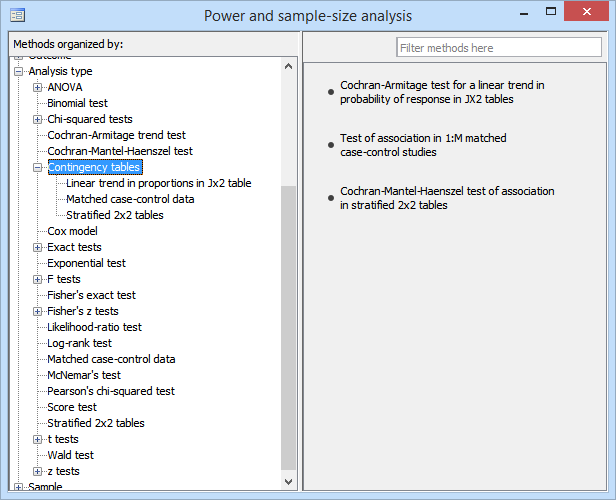• Contingency tables
• Cochran–Mantel–Haenszel test
• Test for trend
• Matched case–control studies
• Survival analysis

### Markov-switching models• Autoregressive model
• Dynamic regression model
• State-dependent parameters
• Transition probabilities
• State membership probabilities

### Survey statistics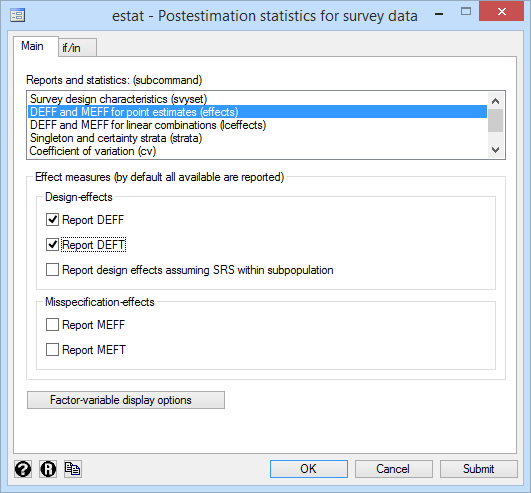### Panel-data survival models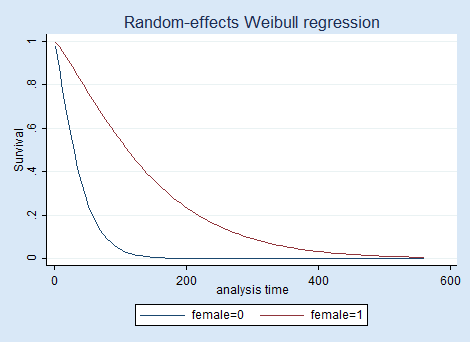• Random effects (intercepts)
• Random coefficients
• Right-censoring
• Exponential, Weibull, ...
• Survival graphs
• More

### Fractional outcome regression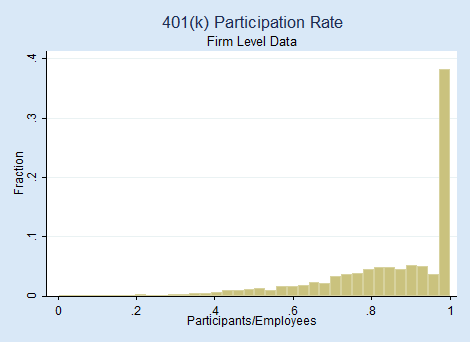• Fractions, proportions, ...
• Beta regression
• Probit and logit
• Heteroskedasticity
• Odds ratios

### Marginal means and marginal effects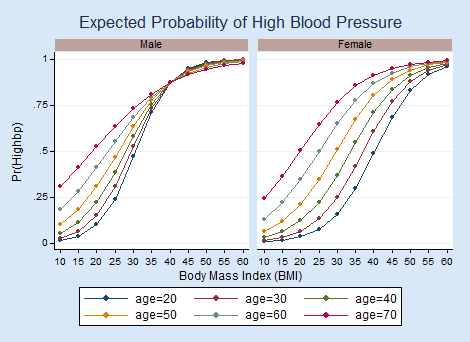### More documentation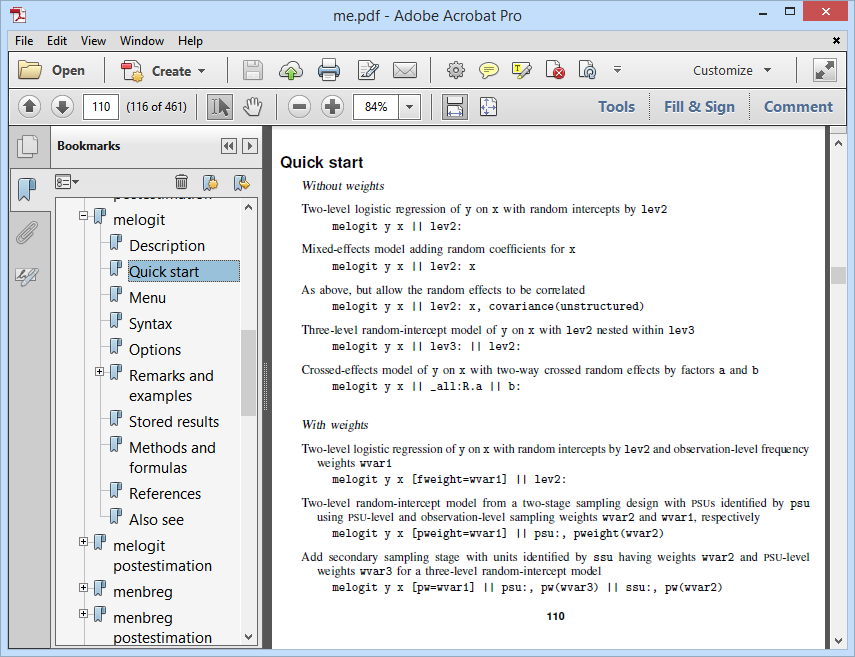• Two all new manuals
• 12,000+ total pages
• Quick starts
• Thousands of worked examples
• Overview of statistical methods
• More

### More statistics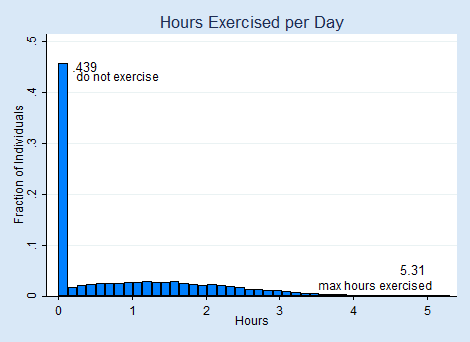### And more